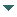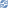# gvSIG

##### Personal tools
You are here: Home Arc
gvSIG DesktopgvSIG Desktop

Cached time 11/21/13 11:24:39# Arc

Up one level
 ◄ Previous Curve Next: Surface ►

An arc can be created from a central point which works like a radius and another two that will be used to determine the beginning and the end of the arc. In the next example, we will create an arc with the point 0,0 for the radius and from the point 1,0 to the 0,1:

```Point centerPoint = geometryManager.createPoint(0, 0, SUBTYPES.GEOM2D);
Point startPoint = geometryManager.createPoint(1, 0, SUBTYPES.GEOM2D);
Point endPoint = geometryManager.createPoint(0, 1, SUBTYPES.GEOM2D);
Arc arc = (Arc)geometryManager.create(TYPES.ARC, SUBTYPES.GEOM2D);
arc.setPoints(centerPoint, startPoint, endPoint);
```

Moreover this way, an arc can be created from a initial point, a radius length and two angles. The next example creates an arc like the created in the previous example, with radius 1 and the beginning on 1,0 (0º) and finnish on 0,1 (90º):

```Point centerPoint = geometryManager.createPoint(0, 0, SUBTYPES.GEOM2D);
Arc arc = (Arc)geometryManager.create(TYPES.ARC, SUBTYPES.GEOM2D);
arc.setPoints(centerPoint, 1, 0, 90);
```
 ◄ Previous Curve Next: Surface ►